# What is a Load Resistor?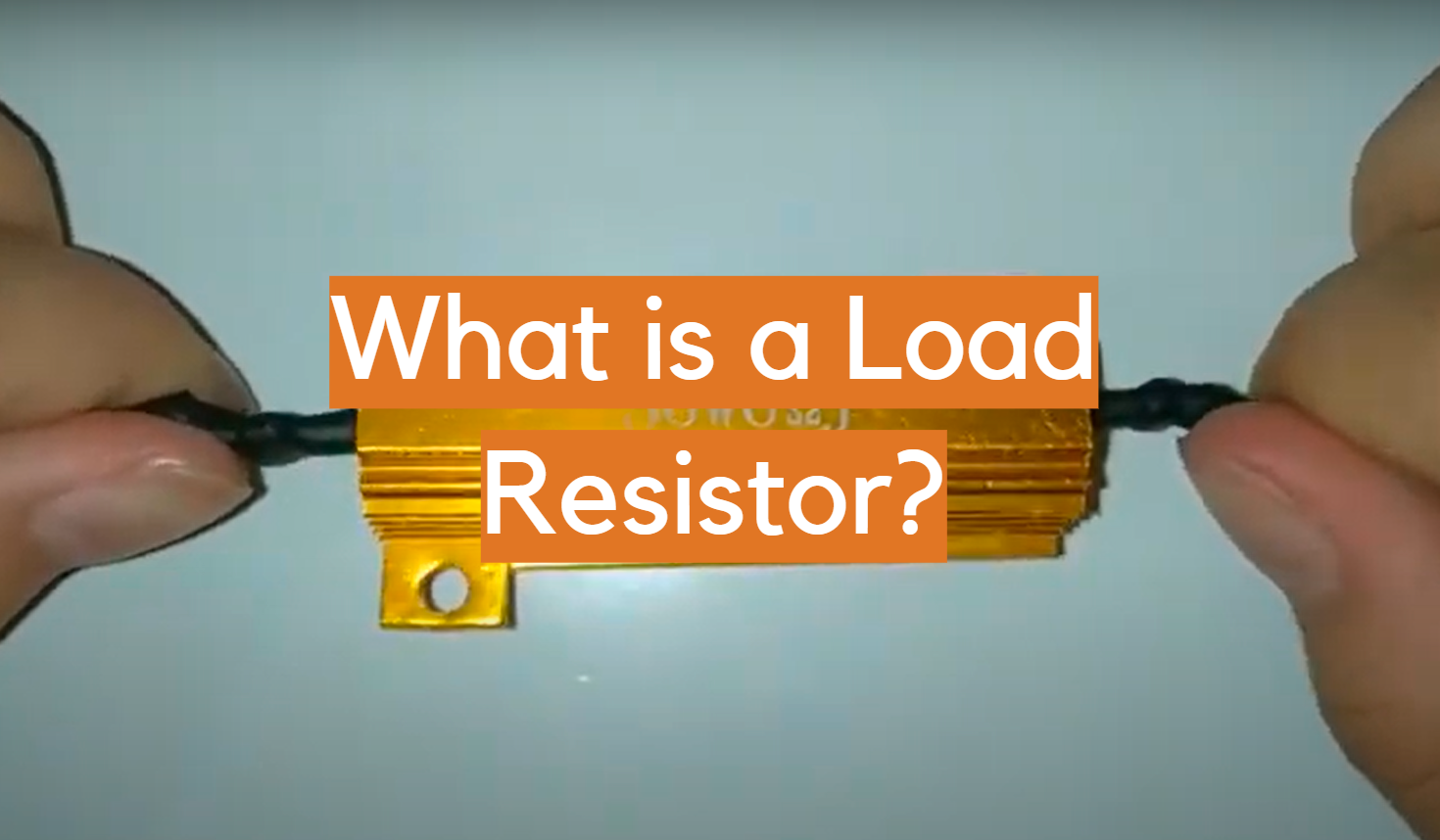A load resistor is an electronic component that limits the amount of current that flows through a circuit. It is important to use a load resistor when you are using high-wattage light bulbs, because they can draw a lot of current and damage the wiring in your home. In this article, we will answer some common questions about load resistors and provide some tips on how to choose the right one for your needs.

In order to understand what a load resistor is, we need to understand the definition of an electrical load. An electrical load is any device in an electrical circuit that consumes energy. The term can be used to refer to either a single appliance such as a light bulb or a motor, or to the total resistive load of an entire circuit.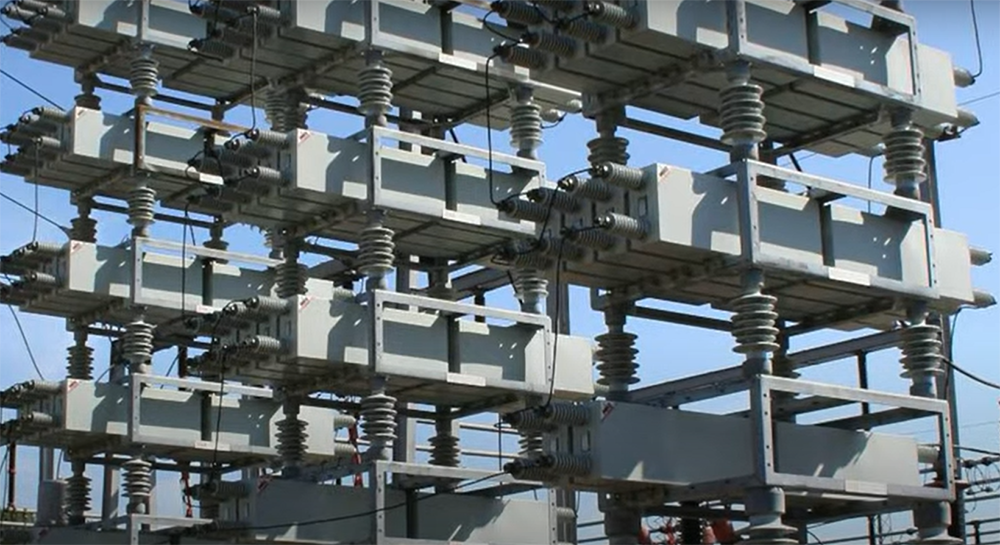Loads are classified as either inductive, capacitive or resistive.

An inductive load is a type of electrical load that consumes only reactive power. In inductive loads, current and voltage values reach their peak at different times which are hard to control and can cause malfunctions in the system. In inductive load current reaches its peak faster than voltage does.

A capacitive load is similar to inductive load in terms and voltage and current values do not match each other. But in capacitive load, voltage reaches its peak value faster than current does. Capacitors are used in a wide variety of electronic applications, such as radios, televisions, computers and power supplies.

A resistive load is made up of devices that have a fixed resistance, such as light bulbs and heating elements. The current through a resistive load is proportional to the voltage applied across it all because the load consumes only active power.

As the current through a resistor increases, so does the heat dissipated by the resistor. This is why you feel heat when you touch an electrical appliance such as a hair dryer or toaster.

Active loads are devices that require active power components to operate. The most common type of active load is a transistor. Transistors can be used as either amplifiers or switches.

Passive loads, on the other hand, do not require a power source to operate. The most common type of passive load is a resistor. Resistors are used to limit the flow of current in a circuit by creating resistance to the flow of electrons. , 

## Definition of a Load Resistor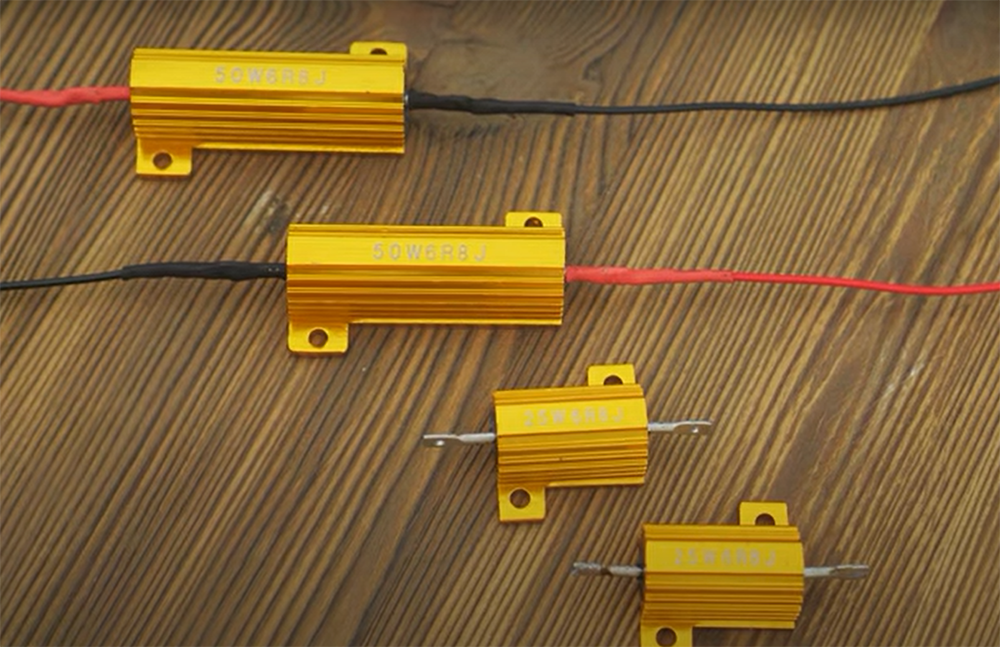A load resistor is a type of resistor that is used with an electronic circuit to create. decrease or increase resistance. Load resistors are used to control the flow of current in a circuit and to protect other components from damage. It typically serves to dissipate excess heat, stabilize the voltage or to prevent electrical surges. By adding resistance to the circuit, they cause a drop in voltage. This is useful when you need to dim LED lights or reduce the power consumption of a device.

There are two main types of load resistors: linear and nonlinear.

Linear load resistors are the most common type of load resistor. They are made of a material that has a linear resistance, such as carbon or metal. This means that the resistance is constant, regardless of the voltage or current passing through them. Linear load resistors are used in circuits where the voltage drop needs to be constant, such as in LED lights. They have a fixed value of resistance, which means they can only be used in specific applications.

Non-linear load resistors are made of a material that has a non-linear resistance, such as semiconductors. This means that the resistance changes with voltage. Non-linear load resistors are used in circuits where the voltage drop needs to be adjustable, such as in power supplies. , , , 

Load resistors are used in a variety of applications, including: rectifiers, inverters, motor starters, switch mode power supplies (SMPS), and more. They are also used in electronic devices such as computers and cell phones to protect against voltage spikes.

As you can see load resistors see a plenty of use in various industries. Let’s take a look at some more applications in detail.

### Impedance matching

In order to maximize the power transfer in a circuit, it is necessary to match the impedances of the load and the source. If the impedances are not matched, some of the power will be reflected back to the source, and this can cause damage to sensitive electronic components.

Load resistors are used to match the impedance of the load with that of the source. By using a resistor with a value that is equal to or greater than the impedance of the load, you can ensure that all of the power is transferred from the source to the load. ,, 

### Use in LED lights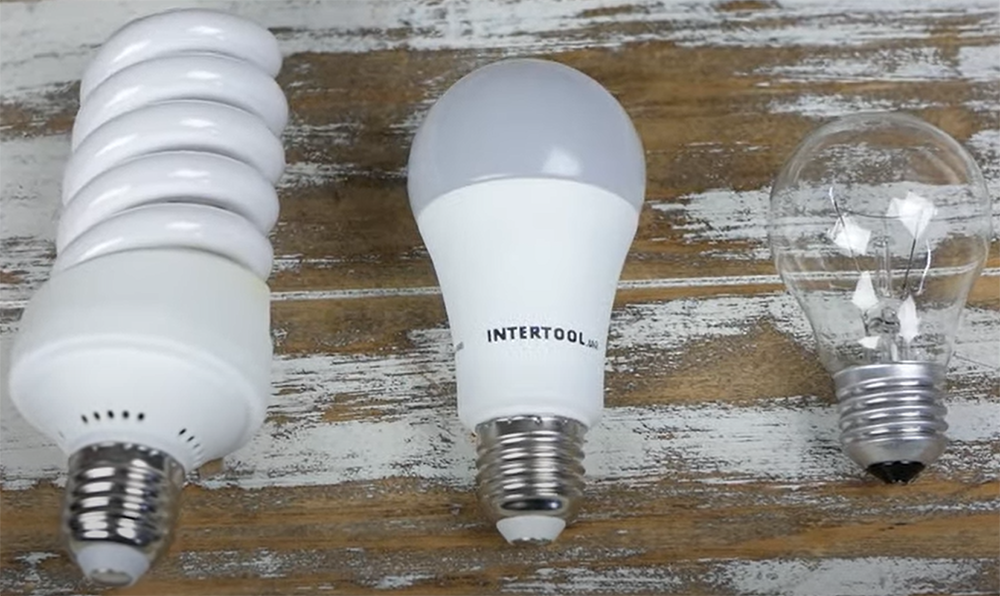One of the most common applications of load resistors is in LED lights. LEDs are designed to operate at a specific voltage, usually around 1.2V. However, the voltage in a car’s electrical system is often higher than this. This means that if you connect an LED directly to the car’s electrical system, it will burn out. To prevent this from happening, you need to use a load resistor in series with the LED. The resistor will lower the voltage to the level that the LED can handle.

Load resistors are also used to dim LED lights. By changing the resistance, you can change the amount of power that flows through the LED, and therefore its brightness.

They also can prevent flickering in LED lights. When an LED is turned on, the current flowing through it causes a voltage drop across the resistor. This voltage drop causes a change in the brightness of the LED, which can cause it to flicker. , 

### Managing excessive electrical energy

Load resistors are used in a number of applications where it is necessary to dissipate excess electrical energy. One common example is in LED lighting, where load resistors are used to prevent damage to the LEDs from voltage surges. 

### Checking Maximum Power Transfer in a Circuit

In electrical engineering, it is often necessary to know the maximum power that can be transferred from one circuit to another. This can be done by using a load resistor in the receiving circuit. By measuring the voltage and current in the circuit, the maximum power transfer can be calculated. , 

### Checking Minimum Current Flow in a Circuit

Another common application of load resistors is to check the minimum current flow in a circuit. This is often done in order to ensure that the circuit is functioning properly. , 

### Stabilizing the Output of a Circuit

Load resistors can also be used to stabilize the output of a circuit. As we mentioned, this is often done in order to prevent damage to the circuit from voltage spikes. 

### Signal Conversion

Load resistors are commonly used in signal conversion. For example, they can be used to convert a current signal to a voltage signal. This is often done in order to make the signal compatible with other devices in the circuit. 

As you can see, load resistors have a wide range of applications. They are an essential component in many electronic circuits. If you need to dissipate excess electrical energy, stabilize voltages, or convert signals, then load resistors are the perfect solution.

## Calculating Power Dissipation of a Load Resistor

One of the most important factors to consider when using load resistors is power dissipation. This refers to the amount of power that is converted to heat by the resistor. The higher the power dissipation, the more heat will be generated.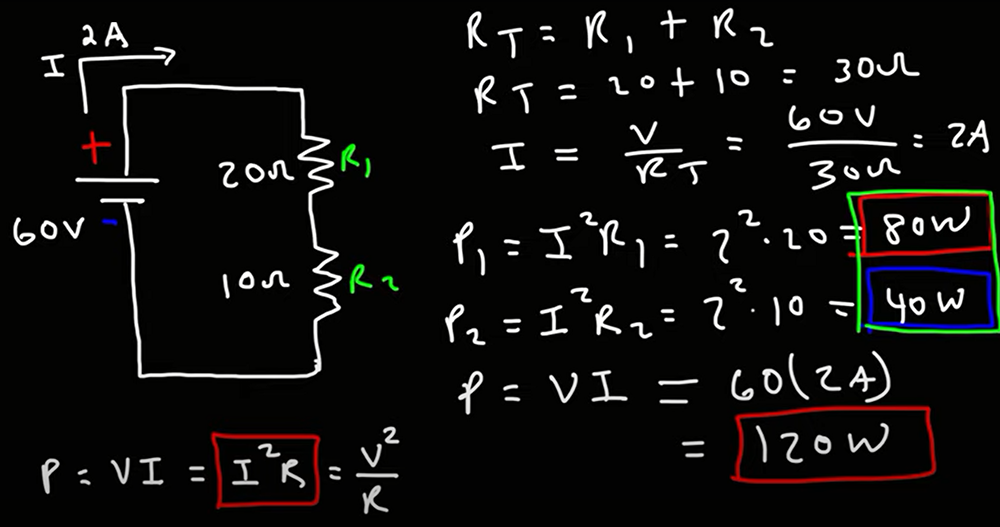In order to calculate the power dissipation of a load resistor, you need to find the voltage and current flowing through it. The power consumption can then be calculated using the following equation:

P = I*V

Where P is the power consumption, I is the current, and V is the voltage. 

For example, if you have a load resistor with a resistance of 100 ohms and a voltage of 12 volts, then the power consumption will be:

P = 12*100

## FAQ

### What is a load resistor in a circuit?

A load resistor is a device that is placed in a circuit to manage resistance. This resistance can be used to control the current in the circuit or to protect other components from excessive currents.

Load resistors are commonly used in electrical circuits where there is a need to control the flow of current. For example, they are often used in light dimmer circuits to control the amount of current flowing through the bulbs. They can also be used in power supplies to limit the amount of current flowing into the devices that they are powering.

Another common use for load resistors is to protect other components from damage that can be caused by excess currents.

### What is the use of load resistors?

Load resistors are used in a variety of applications where it is necessary to dissipate excess power. One common use is in light-emitting diode (LED) circuits, where the load resistor is used to dissipate the excess power that is not converted into light by the LED.

Another common use for load resistors is in voltage regulator circuits. In these circuits, the load resistor is used to dissipate the excess power that is not converted into regulated voltage by the regulator.

### How does a load resistor affect a voltage?

Load resistors affect voltage in a few different ways. Firstly, they can be used to lower the voltage of a circuit. This is useful if you want to run a high-powered device on a lower voltage, for example. Secondly, load resistors can be used to raise the voltage of a circuit. This might be necessary if you’re trying to run a low-powered device on a higher voltage. Finally, load resistors can also be used to change the shape of the voltage waveform. This can be useful for smoothing out spikes or dips in the voltage, for example.

Load resistors are therefore very versatile components that can be used in a variety of different ways to alter the voltage in a circuit.

### What is the difference between a resistor and a load resistor?

A load resistor is merely a type of resistor that is used to dissipate excess current in an electrical circuit and is used for that reason alone.

Load resistors are often used in LED lighting applications because LEDs require less power than traditional incandescent bulbs. By adding a load resistor, the amount of current flowing through the circuit can be controlled, preventing the LEDs from burning out prematurely.

Load resistors can also be used as a voltage divider in a parallel circuit. When used in this way, the load resistor can be used to change the voltage of the entire circuit.

## Conclusion

What are load resistors and how can they improve your circuit? A load resistor is a device that is connected to the output of a circuit. It is simply a resistor that is selected for a purpose of improving output stability and matching impedance. By adding this component, you may be able to resolve common issues with your circuits and achieve better performance. Thanks for reading!